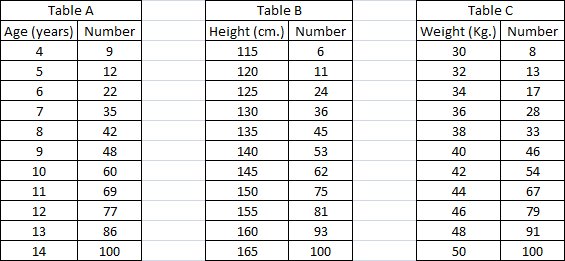### CAT 2003 Question Paper (Leaked) Question 25

Instructions

DIRECTIONS for the following three questions: Answer the questions on the basis of the information given below.Table A below provides data about ages of children in a school. For the age given in the first column, the second column gives the number of children not exceeding the age. For example, first entry indicates that there are 9 children aged 4 years or less. Tables B and C provide data on the heights and weights respectively of the same group of children in a similar format. Assuming that an older child is always taller and weighs more than a younger child, answer the following questions.

Question 25

# Among the children older than 6 years but not exceeding 12 years, how many weigh more than 38 kg.?

Solution

Number of children older than 6 years but not exceeding 12 years are 55 and number older than 12 years are 23 . Also children weighing more than 38 kgs are 67. Out of these 67 , 23 will have age more than 12 . Hence we have 67 - 23 = 44 childrens will the given requirement. Hence option C.